## Example Questions

### Example Question #4 : Rectangles

If a rectangle's width is increased by 20%, and its length is decreased by 20%, which statement most accurately reflects the rectangle's change in area?

The rectangle's area will be reduced by 4%.

The rectangle's area will be decreased by 10.%

The rectangle's area will be increased by 4%.

The rectangle's area will not change.

The rectangle's area will be increased by 10%.

The rectangle's area will be reduced by 4%.

Explanation:

If = A, then we will call the new area B. B results when has been decreased by 20% and has been increased by 20%: in other words, 80% of l and 120% of w.

This is expressed as (0.8) l * (1.2) w = B

The simplest way to determine the result is to create rectangles with actual integers and see what happens. Let's say our first rectangle is a square 10 units by 10 units.

10 * 10 = 100 for our A.

Plugging these numbers into our new equation gives us:

(0.8)10 * (1.2)10 = 96.

So the final step is to compare the areas.  Because we used a 10 x 10 rectangle as our example, this step will be easy, since 100% = 100.

96/100 = 0.96

0.96 * 100 = 96%

Which reflects a 4% reduction in the size of the rectangle.

Any other length and width used for this rectangle will result in a 4% reduction in size when the above parameters are applied.

### Example Question #5 : Rectangles

Amy is building a rectangular patio in her backyard. She plans on making the patio 8 feet wide by 12 feet long. The rectangular bricks she is using are 6 inches long and 4 inches wide. How many bricks will Amy need to build her patio?

384

96

576

424

782

576

Explanation:

Because Amy is building her patio in a rectangle, and the bricks are rectangular, the simplest way to find this solution is to determine how many bricks long and how many bricks wide her patio will be.

To do this, begin by converting the patio's dimensions into inches, since the bricks Amy is using are measured in inches.

Length:

12 feet * 12 inches in a foot = 144 inches long.

Width:

8 feet * 12 inches in a foot = 96 inches wide.

Now, all that's left to do is figure out how many bricks will fit in these spaces.  Let's be intuitive and assume the long sides of the bricks will align with the long sides of the patio.

144 inches long / bricks that are 6 inches long = 24 bricks long

Amy will need columns of 24 bricks to cover the length of her patio.  Now, for the width.

96 inches wide / bricks that are 4 inches wide = 24 bricks wide

Amy will need rows of 24 bricks to cover the width of her patio.

To determine how many bricks Amy will need in total, the last step is to multiply how many rows of bricks she will need by how many columns.

24 bricks to a row * 24 bricks to a column = 576

Amy needs 576 bricks to build her patio.

### Example Question #6 : Rectangles

Quantity A: The area of a rectangle with a perimeter of 40

Quantity B: The area of a triangle with a perimeter of 40

Quantity A is greater

Quantity B is greater

The two quantities are equal

The relationship cannot be determined from the information given

The relationship cannot be determined from the information given

Explanation:

The area of the two shapes depends on their dimensions, which can vary greatly among different combinations. A rectangle with a perimeter of 40 could have dimensions of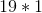(smaller area) or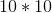(larger area), for instance. Similarly, a triangle could have sides of 10--11--19 (smaller area) or 13--13--14 (larger area). Thus, the relationship cannot be determined without more specific details.

### Example Question #7 : Rectangles

The perimeter of a rectangle is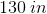. One side is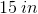. What is the area of this rectangle?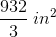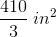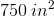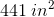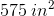Explanation:

Recall that perimeter is defined as: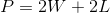For our data, this is: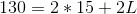Solve for: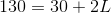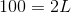Therefore,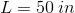This means that the area of the rectangle is equal to: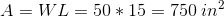### Example Question #8 : Rectangles

Rectangle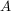has a length of 20 inches and a width of 3 inches. Rectanglehas a length of 9 inches and a width of 10 inches. By what number must the area of rectanglebe multiplied by to equal the area of rectangle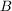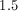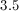Explanation:

Area of rectangleis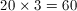.

Area of rectangleis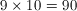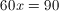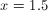Tired of practice problems?

Try live online GRE prep today.